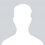# Integration

This rule of integration $\displaystyle\int \limits^{a}_{0}f\left( x\right) dx =\displaystyle\int \limits^{a}_{0}f\left( a - x\right) dx$ I tried by using this rule to solve this integration but it wasn't solve.. the integration is $\displaystyle\int \limits^{\pi }_{0}\frac{x}{2 - \tan \nolimits^{2}\left( x\right) } dx$... I tried wolfram alpha also but it was useless ..... How we can solve this integration by using this rule? . ThanksNote by David Danial
5 years, 10 months ago

This discussion board is a place to discuss our Daily Challenges and the math and science related to those challenges. Explanations are more than just a solution — they should explain the steps and thinking strategies that you used to obtain the solution. Comments should further the discussion of math and science.

When posting on Brilliant:

• Use the emojis to react to an explanation, whether you're congratulating a job well done , or just really confused .
• Ask specific questions about the challenge or the steps in somebody's explanation. Well-posed questions can add a lot to the discussion, but posting "I don't understand!" doesn't help anyone.
• Try to contribute something new to the discussion, whether it is an extension, generalization or other idea related to the challenge.
• Stay on topic — we're all here to learn more about math and science, not to hear about your favorite get-rich-quick scheme or current world events.

MarkdownAppears as
*italics* or _italics_ italics
**bold** or __bold__ bold
- bulleted- list
• bulleted
• list
1. numbered2. list
1. numbered
2. list
Note: you must add a full line of space before and after lists for them to show up correctly
paragraph 1paragraph 2

paragraph 1

paragraph 2

[example link](https://brilliant.org)example link
> This is a quote
This is a quote
    # I indented these lines
# 4 spaces, and now they show
# up as a code block.

print "hello world"
# I indented these lines
# 4 spaces, and now they show
# up as a code block.

print "hello world"
MathAppears as
Remember to wrap math in $$ ... $$ or $ ... $ to ensure proper formatting.
2 \times 3 $2 \times 3$
2^{34} $2^{34}$
a_{i-1} $a_{i-1}$
\frac{2}{3} $\frac{2}{3}$
\sqrt{2} $\sqrt{2}$
\sum_{i=1}^3 $\sum_{i=1}^3$
\sin \theta $\sin \theta$
\boxed{123} $\boxed{123}$

## Comments

Sort by:

Top Newest

you can apply the rule then add the new integral to the original one, and divide by 2. You will be left with the integral:$\displaystyle \frac{\pi}{2} \int_0^{\pi} \frac{1}{2-\tan^2x} dx$.

Did you try that??

- 5 years, 10 months ago

Log in to reply

What will happen if we solve $2 - tan^{2} x = 0$ $x \in (0, \pi)$?.....

- 5 years, 10 months ago

Log in to reply

yes there is discontinueties in the integral at :

$\arctan (\sqrt{2} )$ which is between $\frac{\pi}{4}$ and $\frac{\pi}{2}$.

And $\pi - \arctan (\sqrt{2} )$.

- 5 years, 10 months ago

Log in to reply

So this integration is divergent.... could you please explain more this part of discontinuous.?

- 5 years, 10 months ago

Log in to reply

Yes the integral diverges: lets take take a part of it :

$\displaystyle \int_0^{\arctan (\sqrt{2})} \frac{dx}{2-\tan^2x}$

$\displaystyle = \lim_{a \to \arctan (\sqrt{2})} \int_0^{a} \frac{dx}{2-\tan^2x}$

Use the substitution $u=\tan x$:

$\displaystyle = \lim_{a \to \arctan (\sqrt{2})} \int_0^{\tan a } \frac{du}{(2-u^2)(1+u^2)}$

Easy by applying partial fractions then integrating, then apply the limit and it will yield a value of infinty. So the whole Integral diverges.

- 5 years, 10 months ago

Log in to reply

@Trevor B. You might like this!

Staff - 5 years, 10 months ago

Log in to reply

Yes, the Bounds Trick works nicely here. I know how to get to the indefinite, I just need to make the time to write it down and check the bounds. Partial fracs with what Hasan said originally should make it work.

- 5 years, 10 months ago

Log in to reply

you could try with partial fractions too

- 5 years, 10 months ago

Log in to reply

×

Problem Loading...

Note Loading...

Set Loading...